## Claims from Group A follow a normal distribution with mean 10,000 and standard deviation 1,000. Claims from Group B follow a normal distribu

Question

Claims from Group A follow a normal distribution with mean 10,000 and standard deviation 1,000. Claims from Group B follow a normal distribution with mean 20,000 and standard deviation 2,000. All claim amounts are independent of the other claims. Fifty claims occur in each group. Find the probability the total of the 100 claims exceeds 1,530,000.

in progress 0
2 months 2021-07-23T01:00:52+00:00 1 Answers 10 views 0

0.0287 = 2.87% probability the total of the 100 claims exceeds 1,530,000.

Step-by-step explanation:

Normal Probability Distribution

Problems of normal distributions can be solved using the z-score formula.

In a set with mean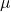and standard deviation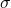, the z-score of a measure X is given by: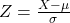The Z-score measures how many standard deviations the measure is from the mean. After finding the Z-score, we look at the z-score table and find the p-value associated with this z-score. This p-value is the probability that the value of the measure is smaller than X, that is, the percentile of X. Subtracting 1 by the p-value, we get the probability that the value of the measure is greater than X.

n instances of a normal variable:

For n instances of a normal variable, the mean is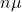and the standard deviation is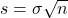Sum of normal variables:

When two normal variables are added, the mean is the sum of the means, while the standard deviation is the square root of the sum of the variances.

Group A follow a normal distribution with mean 10,000 and standard deviation 1,000. 50 claims of group A.

This means that: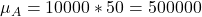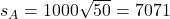Group B follow a normal distribution with mean 20,000 and standard deviation 2,000. 50 claims of group B.

This means that: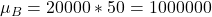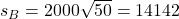Distribution of the total of the 100 claims: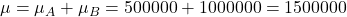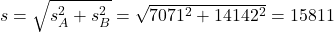Find the probability the total of the 100 claims exceeds 1,530,000.

This is 1 subtracted by the p-value of Z when X = 1530000. SoBy the Central Limit Theorem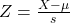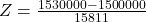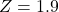has a p-value of 0.9713

1 – 0.9713 = 0.0287

0.0287 = 2.87% probability the total of the 100 claims exceeds 1,530,000.